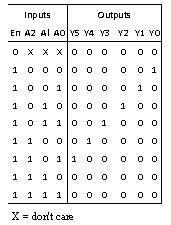Home > VHDL > Logic Circuits > 3 6 Binary Decoder

3:6 Binary Decoder with Enable :

Figure below shows the truth table of 3:6 binary decoder with enable signal.A) First version :

library ieee;

use ieee.std_logic_1164.all;

use ieee.numeric_std.all;

entity decoder3_6 is

port (En: In std_logic;

A: In integer range 0 to 7;

Y: out unsigned (5 downto 0));

end decoder3_6;

architecture behav of decoder3_6 is

begin

process (A)

begin

If (En = '0') then

Y <= "000000";

else

case A is

when 0 => Y <= "000001";

when 1 => Y <= "000010";

when 2 => Y <= "000100";

when 3 => Y <= "001000":

when 4 => Y <= "010000":

when 5 => Y <= "100000";

when others => Y <= "000000";

end case ;

end If ;

end process ;

end behav;

B) Second version :

library ieee;

use ieee.std_logic_1164.all, ieee.numeric_std. all;

entity decoder3_6 is

port (en: in std_logic;

a: in unsigned(2 downto 0);

y: out unsigned(5 downto 0));

end decoder3_6;

architecture behav of decoder3_6 is

begin

process (En, A)

variable enable: unsigned(3 downto 0);

begin

enable := En & A;

case enable is

when "1000" => Y <= "000001";

when "1001" => Y <= "000010";

when "1010" => Y <= "000100";

when "1011" => Y <= "001000";

when "1100" => Y <= "010000";

when "1101" => Y <= "100000";

when others => Y <= "000000";

end case ;

end process ;

end behav;